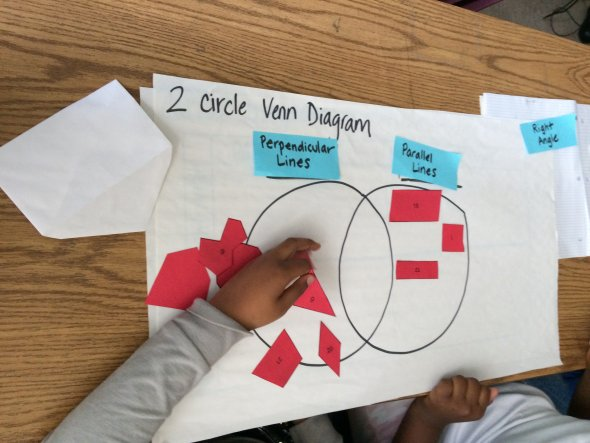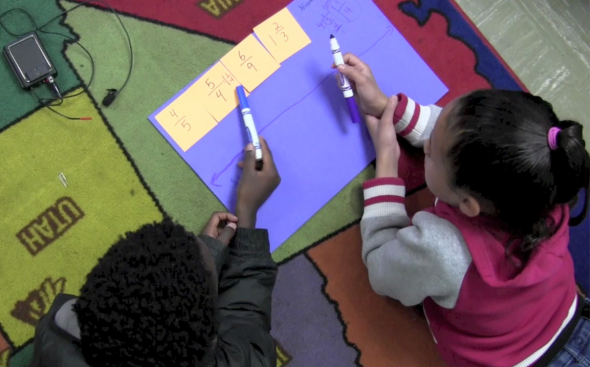In Grade 4, instructional time should focus on three critical areas: developing understanding and fluency with multi-digit multiplication and developing understanding of dividing to find quotients involving multi-digit dividends; developing an understanding of fraction equivalence, addition, and subtraction of fractions with like denominators, and multiplication of fractions by whole numbers; understanding that geometric figures can be analyzed and classified based on their properties, such as having parallel sides, perpendicular sides, particular angle measures, and symmetry.In Grade 5, instructional time should focus on three critical areas: developing fluency with addition and subtraction of fractions, and developing understanding of the multiplication of fractions and of division of fractions in limited cases (unit fractions divided by whole numbers and whole numbers divided by unit fractions); extending division to 2-digit divisors, integrating decimal fractions into the place value system and developing understanding of operations with decimals to hundredths, and developing fluency with whole number and decimal operations; and developing understanding of volume.